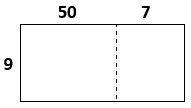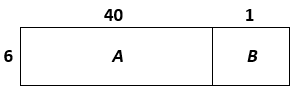# Multi-Digit Multiplication

## Objective

Multiply two-digit numbers by one-digit numbers.

## Common Core Standards

### Core Standards

?

• 4.NBT.B.5 — Multiply a whole number of up to four digits by a one-digit whole number, and multiply two two-digit numbers, using strategies based on place value and the properties of operations. Illustrate and explain the calculation by using equations, rectangular arrays, and/or area models.

?

• 3.NBT.A.3

• 4.NBT.A.1

• 4.NBT.B.4

• 3.OA.B.5

• 3.OA.C.7

## Criteria for Success

?

1. Understand that products can be computed by decomposing numbers into base-ten units, finding partial products of these base-ten units and the multiplier, then adding these partial products together based on the distributive property (e.g., 365 x 4 = (300 x 4) + (60 x 4) + (5 x 4)). (Note that students need not know the term “distributive property.”)
2. Multiply a two-digit whole number by a one-digit whole number using area models and equations.
3. Estimate products by rounding factors to the largest place value.
4. Solve one-step word problems involving multiplication of two-digit by one-digit numbers, understanding that an area model can be used to solve equal groups and comparison problems (MP.4).

## Tips for Teachers

?

• Throughout the remainder of the topic, the main visual model used is the area model. If students seem to be struggling with place value understanding or don’t yet seem ready for the area model for some other reason, you might create a lesson to use before this one that focuses on the use of the base ten block array and/or graph paper array to build understanding toward the area model. This applet Partial Product Finder by the Math Learning Center may be helpful if you take that route. (See Unit-Specific Intellectual Prep section of the Unit Overview for examples of those representations.)
• Before or in place of much of the purely computational tasks on the Problem Set, you could have students play the game “Multiplication Mix-Up” from Building Conceptual Understanding and Fluency Through Games (Grade 5) by the North Carolina Department of Public Instruction.

#### Remote Learning Guidance

If you need to adapt or shorten this lesson for remote learning, we suggest prioritizing Anchor Task 2 (benefits from worked example). Find more guidance on adapting our math curriculum for remote learning here.

#### Fishtank Plus

• Problem Set
• Student Handout Editor
• Vocabulary Package

?

### Problem 1

Mr. Wynn gets some butcher paper from the teacher supply room to put down the length of the hallway. The butcher paper is 3 feet tall and 20 feet long. When he puts it up in the hallway, he realizes he needs a little more to fully cover the wall. The extra piece he needed is 3 feet tall and 3 feet long.

a. How many square feet of butcher block paper did Mr. Wynn put in the hallway?

b. What was the total length of width of the space Mr. Wynn ended up covering?

#### References

EngageNY Mathematics Grade 4 Mathematics > Module 3 > Topic C > Lesson 8Concept Development

Grade 4 Mathematics > Module 3 > Topic C > Lesson 8 of the New York State Common Core Mathematics Curriculum from EngageNY and Great Minds. © 2015 Great Minds. Licensed by EngageNY of the New York State Education Department under the CC BY-NC-SA 3.0 US license. Accessed Dec. 2, 2016, 5:15 p.m..

Modified by The Match Foundation, Inc.

### Problem 2

1. Estimate the product 57 x 9.

2. The area model below represents 57 x 9.a. Label each region of the area model with its respective area.

b. Find the value of 57 x 9. Show your reasoning.

### Problem 3

1. Solve each of the following word problems. Show or explain your work.
1. In the 2017–2018 school year, Match Community Day had 61 students in each grade level. How many students were at Match Community Day that year if the school had Pre-Kindergarten, Kindergarten, and Grades 1–5?
2. A club’s first meeting was attended by 28 people. The first meeting was attended by 4 times as many people as the second meeting. How many people attended the second meeting?
2. Did you use an area model to represent either or both problems above? If not, could you have? Why or why not?

#### References

page 9

From EngageNY.org of the New York State Education Department. New York State Testing Program Grade 4 Common Core Mathematics Test Released Questions with Annotations August 2013. Internet. Available from https://www.engageny.org/resource/new-york-state-common-core-sample-questions/file/103191; accessed March 23, 2018, 3:44 p.m..

Modified by The Match Foundation, Inc.

## Problem Set & Homework

#### Discussion of Problem Set

• Look at #2d. How can you use rounding to assess the reasonableness of your answer? How can you use what you know about multiples of 9 to further assess your answer’s reasonableness?
• The model used in #3 is different from the ones we’ve seen so far. How is it similar to and different from an area model? Which answers were you able to eliminate right away?
• Can you use an area model for #4–#6? Why or why not?
• Did you use any strategies from Lesson 11 to solve any problems on today’s Problem Set? For example, #7c or #7d?

?

### Problem 1

In the area model shown, what are the values of A and B?### Problem 2

Solve. Show or explain your work.

35 x 7

### Problem 3

A countertop in a kitchen measures 4 feet deep by 26 feet long. What is the area, in square feet, of the countertop?

### Mastery Response

?